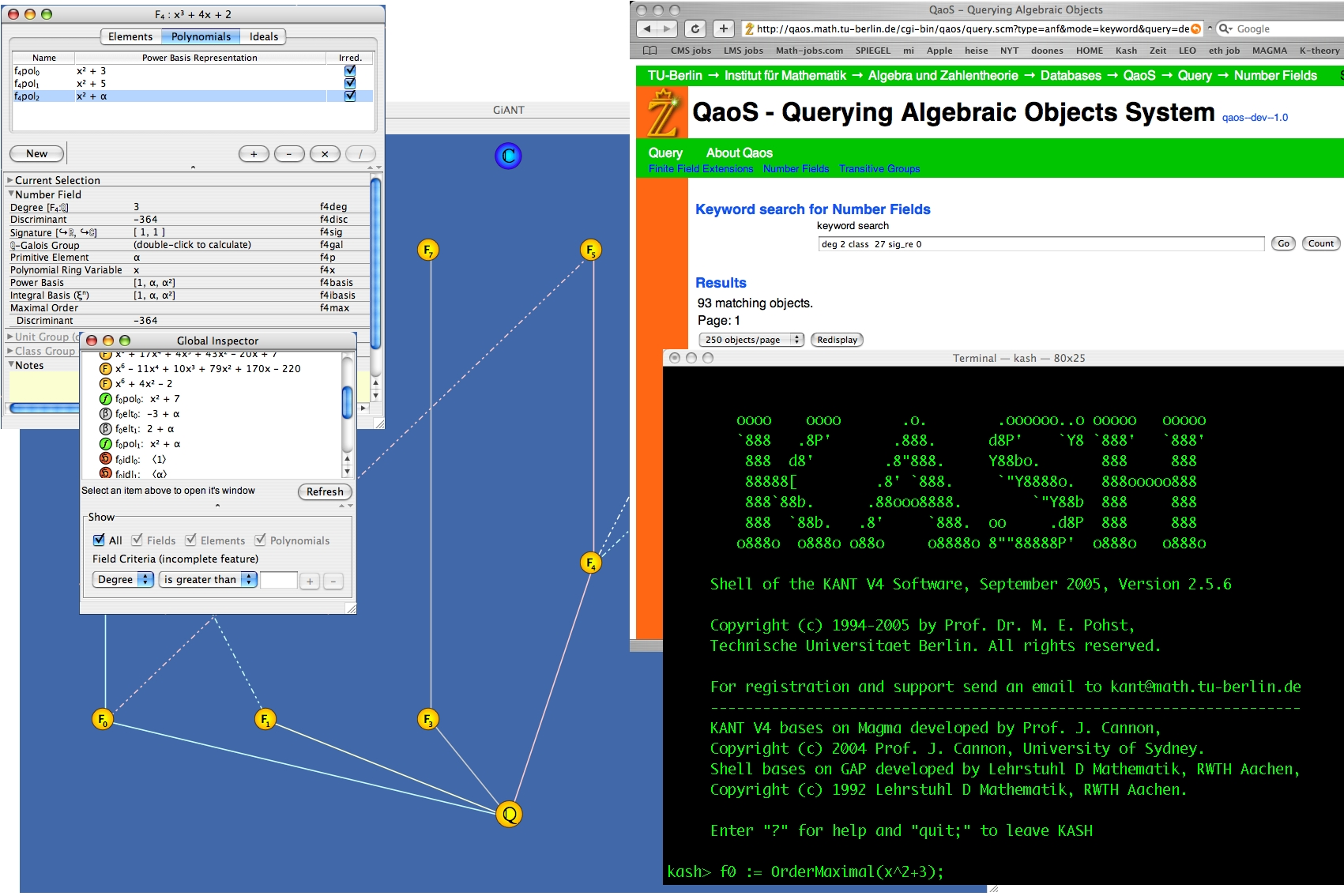# KANT / KASH

### Computational Algebraic Number Theory / KAnt SHell

The KANT Group: [members] [publications] [database] [links] - [Institut für Mathematik, TU Berlin]

KANT is a software package for mathematicians interested in algebraic number theory. For those KANT is a tool for sophisticated computations in number fields, in global function fields, and in local fields.

Many parts of KASH have been redesigned since the release of KASH 2.5. We must apologize to our old KASH users that code written for KASH 2.x will not run under KASH3 without modifications. There still will be bug fixes and at least one more version of KASH 2.x.The main new features of KASH3 are

• modular type system,
• integrated help system with inline help and a reference manual in html format,
• local fields (unramified extensions, ramified extensions polynomial factorization),
• access to the QaoS databases of numberfields and transitive groups,
• new generic function names.

KASH is freely available. You can pick up the current release of KASH using ftp. You can download the documentation for KASH separately. Most of the functionality of KASH is also available through the web interface webkash. Many of the algorithms, which are implemented in KASH/KANT, are described in the publications of the KANT Group. Take a look at a KASH sample-session (taken from the ICM 98 Mathematical Software Session).

Please mail all your questions, suggestions, comments and bug reports concerning KASH to kant@math.tu-berlin.de.

## The main features of the current release are:

### Computations in number fields

• arithmetic of algebraic numbers,
• computation of maximal orders in number fields,
• modular computation of resultants,
• unconditional and conditional (GRH) computation of class groups of number fields,
• unconditional and conditional (GRH) computation of fundamental units in arbitrary orders,
• S-unit computation,
• computation of all subfields of a number field,
• determination of Galois groups of number fields up to degree 15,
• ray class groups,
• automorphisms of normal and abelian fields,
• computation of minimal vectors and eutactic coefficients for quadratic fields

### Ideals in number fields

• arithmetic of fractional ideals in number fields,
• computation of prime ideal decompositions of fractional ideals in number fields,
• (ray) class group representation of an ideal (discrete logarithm for ray class groups),
• computation of the multiplicative group of residue rings of maximal orders modulo ideals and infinite primes,
• Chinese remainder for ideals and infinite places,

### Relative extensions of number fields

• computation of maximal orders (relative Round 2),
• arithmetic of algebraic numbers,
• signature of polynomials,
• normal forms of modules in relative extensions,
• arithmetic of relative ideals,
• computation of a 2-element-representation for relative ideals,
• Kummer extensions of prime degree, relative field discriminant and integral basis,

### Computations in class field theory

• Hilbert and ray class fields of imaginary quadratic fields by complex multiplication,
• Hilbert class fields of totally real number fields via the computation of Stark units by Stark's conjecture,
• computation of discriminants and conductors of ray class fields,
• computation of ray class fields, subgroups of ray class groups supported via Kummer theory,
• Artin map and automorphisms of ray class fields,

### Galois groups

• computation of Galois groups of polynomials over Q up to degree 21 and their representation as permutation groups on the roots,
• symbolic computation of Galois groups of polynomials over Q, number fields and Q(x) up to degree 7,

### Lattices

• lattices and enumeration of lattice points,
• lattices and lattice reduction for lattices over numberfields,

### Diophantine equations

• Thue equation solver,
• unit equations and exceptional units,
• index form equations,
• integral points on Mordell curves,
• norm equation solver for absolute and relative extensions,

### Algebraic function fields over finite fields, Q or number fields

• arithmetic of algebraic functions,
• genus computation,
• places, divisors and Riemann-Roch spaces,
• dimension of exact constant field,
• computation of maximal orders in function fields,
• arithmetic of fractional ideals of orders of function fields,
• computation of prime ideal decompositions of fractional ideals,
• basis reduction for orders, fundamental unit computation in global function fields,
• determination of places of degree one in global function fields,
• divisor reduction, divisor class representation
• S-units for global function fields
• differentials, differential spaces, differentiations
• Cartier operator for global function fields
• Hasse-Witt invariant of a global function field
• L-polynomial computation for global function fields
• gap numbers, Weierstrass places
• strong approximation
• basic functions for drinfeld modules

### Specials

• factorization of polynomials over number fields,
• basic linear algebra over number fields,
• a package for Abelian groups,

### Environment

• Pascal like programming language,
• user extensible library,
• help system (TeX based online help),
• more than 1000 pages of documentation,
• examples for the programming language,
• PVM access in the programming language of KASH,
• PVM support for many parts of KANT,
• database for number theoretical data,
• colour support.